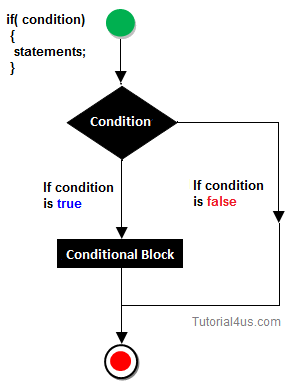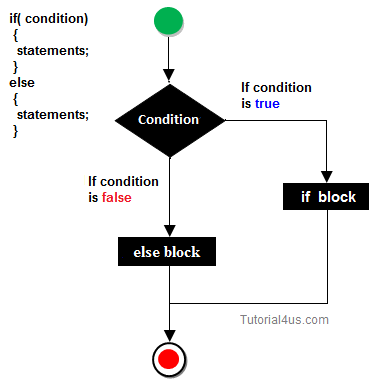# If else Statement

## If else Statement

The if statement is used in JavaScript to execute the code if condition is true or false. There are three forms of if statement.

• If Statement
• If else statement
• if else if statement

## JavaScript If statement

if is most basic statement of Decision making statement. It tells to program to execute a certain part of code only if particular condition or test is true.Syntax

## Syntax

```if(expression)
{
//set of statements
}
```

## Example

```<script>
var a=10;
if(a>5)
{
document.write("value of a is greater than 5");
}
</script>
```

## JavaScript if-else statement

In general it can be used to execute one block of statement among two blocks.## Syntax

```if(expression)
{
//set of statements
}
else
{
//set of statements
}
```

## Example of if..else statement

```<script>
var a=40;
if(a%2==0)
{
document.write("a is even number");
}
else{
document.write("a is odd number");
}
</script>
```

a is even number

## JavaScript If...else if statement

It evaluates the content only if expression is true from several expressions.

## Syntax

```if(expression1)
{
//content to be evaluated if expression1 is true
}
else if(expression2)
{
//content to be evaluated if expression2 is true
}
else
{
//content to be evaluated if no expression is true
}
```

## Example of if..else if statement

```<script>
var a=40;
if(a==20)
{
document.write("a is equal to 20");
}
else if(a==5)
{
document.write("a is equal to 5");
}
else if(a==30)
{
document.write("a is equal to 30");
}
else
{
document.write("a is not equal to 20, 5 or 30");
}
</script>
```

a is equal to 40